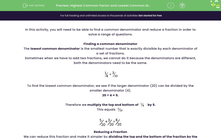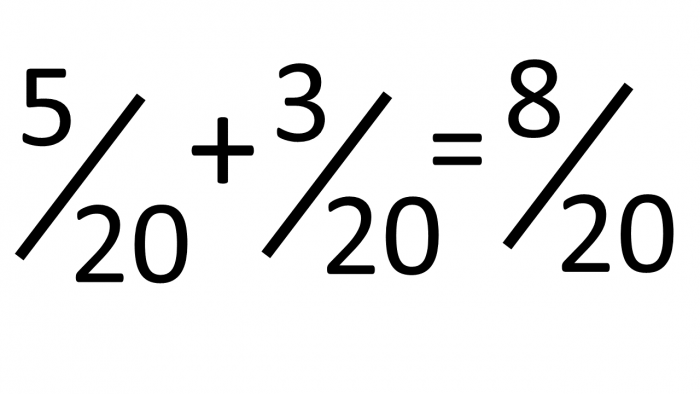# Highest Common Factor and Lowest Common Multiple

In this worksheet, students will be asked to recap their knowledge of common denominators, reducing fractions and then solving worded problems.Key stage:  KS 2

Curriculum topic:   Number: Fractions, Decimals and Percentages

Curriculum subtopic:   Use Common Factors

Difficulty level:#### Worksheet Overview

In this activity, you will need to be able to find a common denominator and simplify a fraction in order to solve a range of questions.

Finding a common denominator

The lowest common denominator is the smallest number that is exactly divisible by each denominator of a set of fractions.

Sometimes when we have to add two fractions, we cannot do it because the denominators are different, both the denominators need to be the same.To find the lowest common denominator, we see if the larger denominator (20) can be divided by the smaller denominator (4).

20 ÷ 4 = 5.

Therefore we multiply the top (numerator) and bottom (denominator) ofby 5.

This equalsSimplifying a Fraction

We can simplify this fraction by dividing the numerator and the denominator by the same number.

We want to simplify a fraction to its lowest terms, so we divide the numerator and the denominator by their highest common factor.

The highest number that goes into 8 and 20 is 4.

8 ÷ 4 = 2

20 ÷ 4 = 5

ThereforeOver to you now to use this knowledge to solve a range of different questions.

### What is EdPlace?

We're your National Curriculum aligned online education content provider helping each child succeed in English, maths and science from year 1 to GCSE. With an EdPlace account you’ll be able to track and measure progress, helping each child achieve their best. We build confidence and attainment by personalising each child’s learning at a level that suits them.

Get started